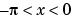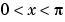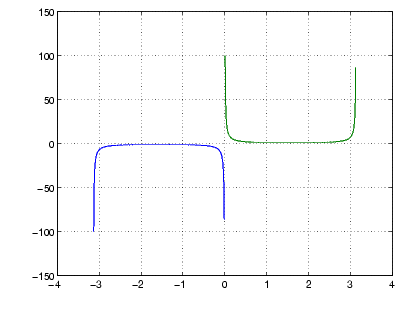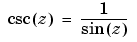MATLAB Function Referencecsc

Cosecant of an argument in radians

Syntax

• ```Y = csc(x)
```

Description

The `csc` function operates element-wise on arrays. The function's domains and ranges include complex values. All angles are in radians.

```Y = csc(x) ``` returns the cosecant for each element of `x`.

Examples

Graph the cosecant over the domainsand.

• ```x1 = -pi+0.01:0.01:-0.01;
x2 = 0.01:0.01:pi-0.01;
plot(x1,csc(x1),x2,csc(x2)), grid on```

Definition

The cosecant can be defined as

•Algorithm

`csc` uses FDLIBM, which was developed at SunSoft, a Sun Microsystems, Inc. business, by Kwok C. Ng, and others. For information about FDLIBM, see http://www.netlib.org.

See Also

`acsc`, `acsch`, `cscd`, `csch`

© 1994-2005 The MathWorks, Inc.